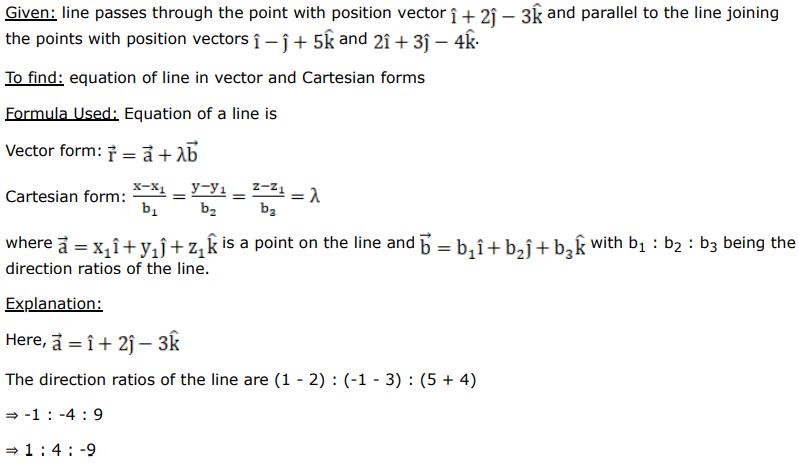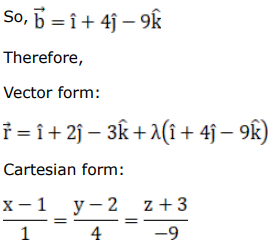# Find the vector equation of a line passing through the point having the position vector

Question:

Find the vector equation of a line passing through the point having the position vector $(\hat{i}+2 \hat{j}-3 \hat{k})$ and parallel to the line joining the points with position vectors $(\hat{\mathrm{i}}-\hat{\mathrm{j}}+5 \hat{\mathrm{k}})$ and $(2 \hat{\mathrm{i}}+3 \hat{\mathrm{j}}-4 \hat{\mathrm{k}})$.

Also, find the Cartesian equivalents of this equation.

Solution: